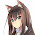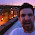## Monday, April 6, 2015

### Mathematical APIs

You can use mathematical APIs to smooth the onboarding process for new users of your library by reusing their existing intuition for algebraic operations.

Let's use an example from my `turtle` library for shell scripting, which uses the `Shell Text` type to represent a stream of lines. You can forward such a stream to the console using `stdout`:

``stdout :: Shell Text -> IO ()``

Thanks to Haskell's `OverloadedStrings` extension, we can use a string literal to encode a 1-element stream that emits just that one string literal:

``````>>> :set -XOverloadedStrings
>>> import Turtle
>>> stdout "Hello, world!"
Hello, world
>>>``````

Now let's drop a thin mathematical API on top of these streams so that we can treat streams like ordinary numbers. These examples will only work with `turtle-1.1.0` and later.

If we add streams, we concatenate their elements in order. Here we concatenate three 1-element streams to generate a combined 3-element stream:

``````>>> stdout ("Line 1" + "Line 2" + "Line 3")
Line 1
Line 2
Line 3
>>>``````

`0` will be the empty stream:

``````>>> stdout 0
>>> -- This prints nothing``````

`1` is a stream that emits a single empty string:

``````>>> stdout 1

>>>``````

So what is 2? Well, it stands to reason that 2 must be 1 + 1:

``````>>> stdout 2

>>> stdout (1 + 1) -- Same thing

>>>``````

Similarly, 3 must be 1 + 1 + 1:

``````>>> stdout 3

>>>``````

... and so forth.

If we multiply two 1-element streams, we generate a new 1-element stream that concatenates their strings.

``````>>> stdout ("123" * "456")
123456
>>>``````

Now what do you think will happen if I do this?

``````>>> let x = "Line 1" + "Line 2"  -- A stream with two elements
>>> let y = "Line A" + "Line B"  -- A stream with two elements
>>> stdout (x * ", " * y)        -- What this will print?``````

First we substitute `x` and `y` with their definitions:

``````x * ", " * y

= ("Line 1" + "Line 2") * ", " * ("Line A" + "Line B")``````

Then we expand out the multiplication to get four terms:

``````= "Line 1" * ", " * "Line A"
+ "Line 1" * ", " * "Line B"
+ "Line 2" * ", " * "Line A"
+ "Line 2" * ", " * "Line B"``````

Then we use the rule that multiplying 1-element streams just concatenates their strings:

``````= "Line 1, Line A"
+ "Line 1, Line B"
+ "Line 2, Line A"
+ "Line 2, Line B"``````

... and there's our final result! We expect our stream to have four elements, one for each permutation of elements from the left and right streams.

Let's verify that:

``````>>> stdout (x * ", " * y)
Line 1, Line A
Line 1, Line B
Line 2, Line A
Line 2, Line B``````

We can even leverage our algebraic intuition when working with impure streams:

``````>>> stdout (stdin * ("!" + "?"))
Test<Enter>
Test!
Test?
ABC<Enter>
ABC!
ABC?
...``````

This is why I love mathematical APIs: they are concise, general, and expressive.

People judge programming languages using mindshare, but the mindshare of mathematics dwarfs all programming languages combined. By specifying programs mathematically we can unlock a large and latent pool of programming talent.

1.Operations (+) and (*) should be reserved for number-like stuff (rings or some of their generalizations). Using it for streams is very confusing as not many interesting laws hold here (ultimately this stems from (+) not being commutative).

I give you that it does seem like a clever idea initially, but eventually it fails precisely because there is no mathematical structure here (kind of ironic given the title of this post).

It very much reminds me of using instances (e.g. for Monad) for stuff that is not because it fails some of the laws. I think not many people bother to check the laws actually. They assume that if it binds like a duck and returns like a duck, it must be a duck. But does it associate like the duck? That's the real question!

1.I don't understand what you mean when you say there are not many interesting laws. (+) is associative and its identity is 0. (*) is associative and its identity is 1. (*) right-distributes over (+).

2.These streams seem to be ordered though, which would mean that a + b != b + a and that violates commutativity. Of course, that's just because we haven't defined the equivalence relation as one that doesn't care about the order of elements in the stream. Intuitively, all we can go on is that the outcomes are possible to distinguish some way, ie that there is a valid equivalence relation for which + does not commute.

3.These streams seem to be ordered though, which would mean that a + b != b + a and that violates commutativity. Of course, that's just because we haven't defined the equivalence relation as one that doesn't care about the order of elements in the stream. Intuitively, all we can go on is that the outcomes are possible to distinguish some way, ie that there is a valid equivalence relation for which + does not commute.